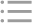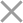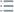## Fill out the form in 2 simple steps below:

Step 1

Step 2
** By clicking this button you agree to our Privacy Policy statementRequest our prices
--Select currency--
USD
EUR
* By clicking this button you agree to our Privacy Policy statementFree PVS‑Studio license for Microsoft MVP specialists
* By clicking this button you agree to our Privacy Policy statementTo get the licence for your open-source project, please fill out this form
* By clicking this button you agree to our Privacy Policy statementI am interested to try it on the platforms:
 Windows Linux macOS PVS-Studio for .NET Core JetBrains Rider
* By clicking this button you agree to our Privacy Policy statementMessage submitted.

Your message has been sent. We will email you at

check your Spam/Junk folder and click the "Not Spam" button for our message.
This way, you won't miss messages from our team in the future.

>
>
>
V6096. An odd precise comparison. Consi…Introduction
Analyzing projects
On Windows
On Linux and macOS
Continuous use of the analyzer in software development
Deploying the analyzer in cloud Continuous Integration services
Managing analysis results
Analyzer diagnostics
General Analysis (C++)
General Analysis (C#)
General Analysis (Java)
Diagnosis of micro-optimizations (C++)
Diagnosis of micro-optimizations (C#)
Diagnosis of 64-bit errors (Viva64, C++)
Customer specific requests (C++)
MISRA errors
AUTOSAR errors
OWASP errors (C++)
OWASP errors (C#)
OWASP errors (Java)
Problems related to code analyzerContents

# V6096. An odd precise comparison. Consider using a comparison with defined precision: Math.abs(A - B) < Epsilon or Math.abs(A - B) > Epsilon.

Oct 09 2020

The analyzer has detected a suspicious code fragment where floating-point numbers are compared using operator '==' or '!='. Such code may contain a bug.

Let's discuss an example of correct code first:

``````double a = 0.5;
if (a == 0.5) //ok
++x;``````

This comparison is correct. Before executing it, the 'a' variable is explicitly initialized to value '0.5', and it is this value the comparison is done over. The expression will evaluate to 'true'.

So, strict comparisons are permitted in certain cases - but not all the time. Here's an example of incorrect code:

``````double b = Math.sin(Math.PI / 6.0);
if (b == 0.5) //err
++x;``````

The 'b == 0.5' condition proves false because the 'Math.sin(Math.PI / 6.0)' expression evaluates to 0.49999999999999994. This number is very close but still not equal to '0.5'.

One way to fix this is to compare the difference of the two values against some reference value (i.e. amount of error, which in this case is expressed by variable 'epsilon'):

``````double b = Math.sin(Math.PI / 6.0);
if (Math.abs(b - 0.5) < epsilon) //ok
++x;``````

You should estimate the error amount appropriately, depending on what values are being compared.

The analyzer points out those code fragments where floating-point numbers are compared using operator '!=' or '==', but it's the programmer alone who can figure out whether or not such comparison is incorrect.

References:

This diagnostic is classified as: